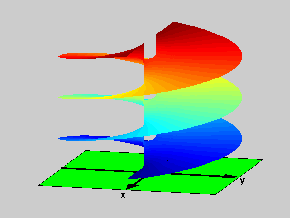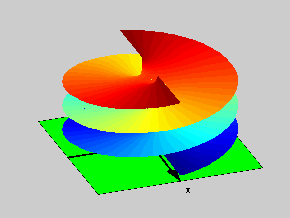18.04 | Fall 1999 | Undergraduate

# Complex Variables with Applications

Study Materials

## Riemann Surfaces: f(z)=log(z2-1)

(All images created with MATLAB® software)

Click on pictures for more information and an image of higher resolution.Figure 1: Riemann Surface of the function f(z)=log(z2-1)

To construct the Riemann Surface for the function f(z)=log(z2-1), we start with the complex plane with two cuts along the real axis: one for x > 1 and the other for x < -1. Here the function has infinitely many branches. Then we proceed in the same fashion used in the previous examples. The two pictures show two views of the resulting surface. We note that this Riemann Surface is an object in three dimensional space. Furthermore, this is an example with three branch points, in which it differs from all the prior examples (which only had two branch points). The branch points are z=1, z=2 and infinity.Figure 2: Top view for the Riemann Surface of the function f(z)=log(z2-1)